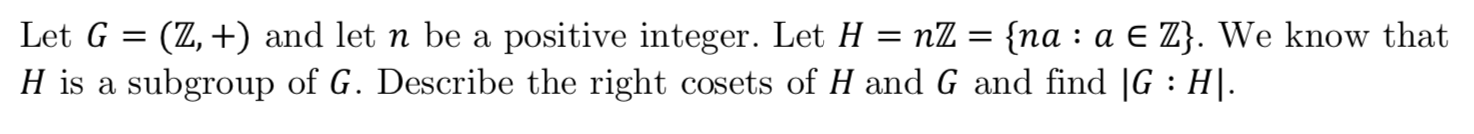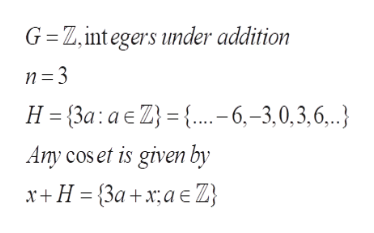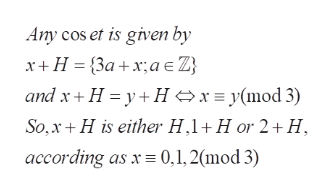# Let G (Z,+) and let n be a positive integer. Let H nZ = {na : a E Z}. We know thatH is a subgroup of G. Describe the right cosets of H and G and find |G H.

Questionhelp_outlineImage TranscriptioncloseLet G (Z,+) and let n be a positive integer. Let H nZ = {na : a E Z}. We know that H is a subgroup of G. Describe the right cosets of H and G and find |G H. fullscreen
check_circleExpert Solution
Step 1

To determine (to list the elements of) the right cosets of the given subgroup

Step 2

Now, Z is an abelian group , so the right cosets are the same as the left cosets. (every subgroup is normal). Let us first consider an example, say n =3 and this generalizes to all values of nhelp_outlineImage TranscriptioncloseG Z, int egers under addition H {3a: a E Z {..-6,-3,0,3,6,.. Any cos et is given by x+H 3a+xa } fullscreen
Step 3

The coset x+H is determined by the equival...help_outlineImage TranscriptioncloseAny cos et is given by x+H {3ax;ae Z and x H yHxy(mod 3) So,x H is either H,1 H or 2+H according as x 0,1, 2(mod 3) fullscreen

### Want to see the full answer?

See Solution

#### Want to see this answer and more?

Solutions are written by subject experts who are available 24/7. Questions are typically answered within 1 hour*

See Solution
*Response times may vary by subject and question
Tagged in

### Math